3.5 Binary Indexed Tree

# 树状数组 Binary Indexed Tree (二叉索引树) #

## 一. 一维树状数组概念 #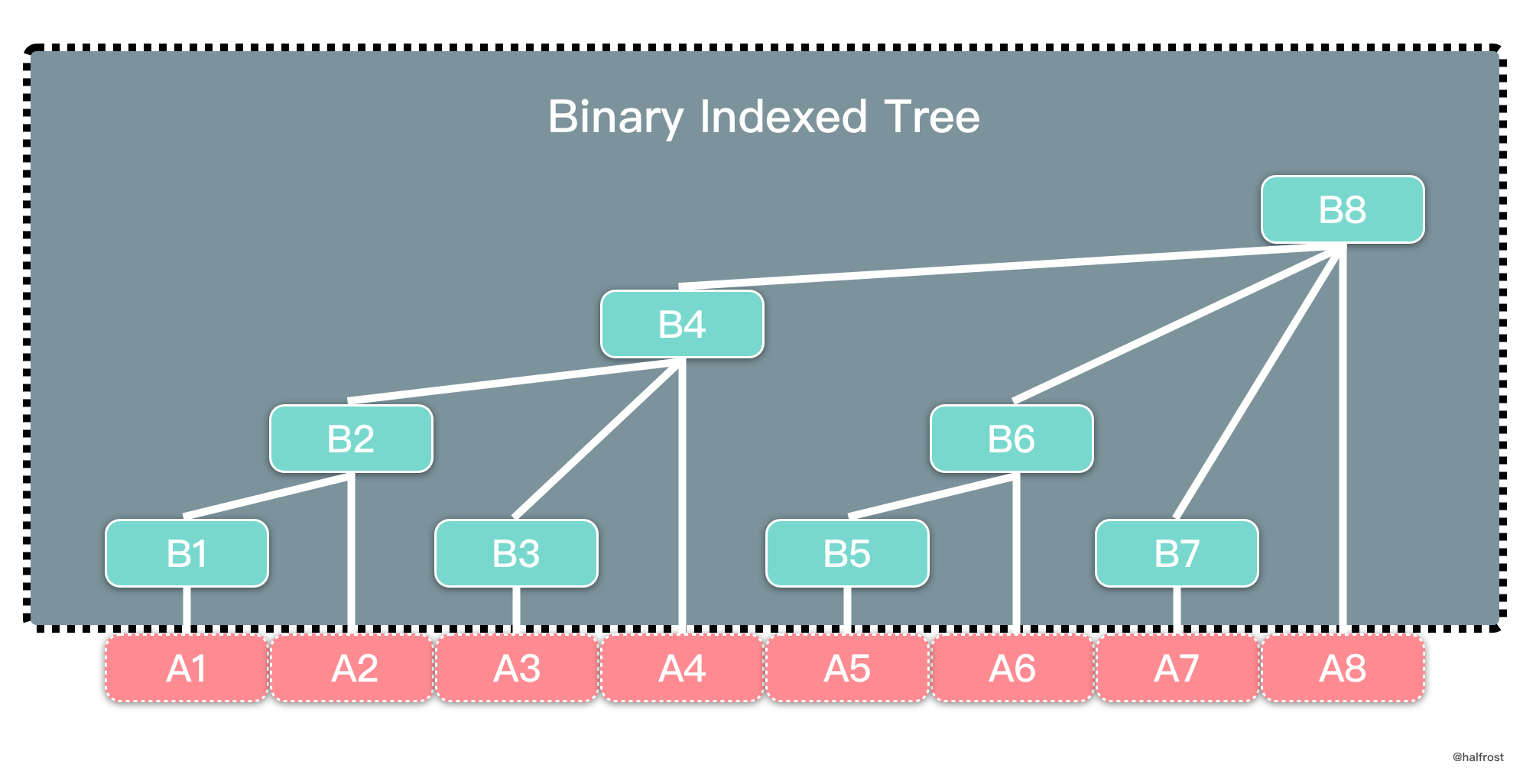### 1. 节点意义 #

\begin{aligned} B_{1} &= A_{1} \\ B_{2} &= B_{1} + A_{2} = A_{1} + A_{2} \\ B_{3} &= A_{3} \\ B_{4} &= B_{2} + B_{3} + A_{4} = A_{1} + A_{2} + A_{3} + A_{4} \\ B_{5} &= A_{5} \\ B_{6} &= B_{5} + A_{6} = A_{5} + A_{6} \\ B_{7} &= A_{7} \\ B_{8} &= B_{4} + B_{6} + B_{7} + A_{8} = A_{1} + A_{2} + A_{3} + A_{4} + A_{5} + A_{6} + A_{7} + A_{8} \\ \end{aligned}

$B_{i} = \sum_{j = i - 2^{k} + 1}^{i} A_{j}$

// BinaryIndexedTree define
type BinaryIndexedTree struct {
tree     []int
capacity int
}

// Init define
func (bit *BinaryIndexedTree) Init(nums []int) {
bit.tree, bit.capacity = make([]int, len(nums)+1), len(nums)+1
for i := 1; i <= len(nums); i++ {
bit.tree[i] += nums[i-1]
for j := i - 2; j >= i-lowbit(i); j-- {
bit.tree[i] += nums[j]
}
}
}


lowbit(i) 函数返回 i 转换成二进制以后，末尾最后一个 1 代表的数值，即 $$2^{k}$$ ，k 为 i 末尾 0 的个数。我们都知道，在计算机系统中，数值一律用补码来表示和存储。原因在于，使用补码，可以将符号位和数值域统一处理；同时，加法和减法也可以统一处理。利用补码，可以 O(1) 算出 lowbit(i)。负数的补码等于正数的原码每位取反再 + 1，加一会使得负数的补码末尾的 0 和正数原码末尾的 0 一样。这两个数进行 & 运算以后，结果即为 lowbit(i)：

func lowbit(x int) int {
return x & -x
}


$(0010 0010)_{2} \& (1101 1110)_{2} = (0000 0010)_{2}$

lowbit(34) 结果是 $$2^{k} = 2^{1} = 2$$

### 2. 插入操作 #

// Add define
func (bit *BinaryIndexedTree) Add(index int, val int) {
for index <= bit.capacity {
bit.tree[index] += val
index += lowbit(index)
}
}


### 3. 查询操作 #

\begin{aligned} Query(i) &= A_{1} + A_{2} + ...... + A_{i} \\ &= A_{1} + A_{2} + A_{i-2^{k}} + A_{i-2^{k}+1} + ...... + A_{i} \\ &= A_{1} + A_{2} + A_{i-2^{k}} + B_{i} \\ &= Query(i-2^{k}) + B_{i} \\ &= Query(i-lowbit(i)) + B_{i} \\ \end{aligned}

$$B_{i}$$ 是树状数组存的值。Query 操作实际是一个递归的过程。lowbit(i) 表示 $$2^{k}$$ ，其中 k 是 i 的二进制表示中末尾 0 的个数。i - lowbit(i) 将 i 的二进制中末尾的 1 去掉，最多有 $$log(i)$$ 个 1，所以查询操作最坏的时间复杂度是 O(log n)。查询操作实现代码如下：

// Query define
func (bit *BinaryIndexedTree) Query(index int) int {
sum := 0
for index >= 1 {
sum += bit.tree[index]
index -= lowbit(index)
}
return sum
}


## 二. 不同场景下树状数组的功能 #

### 1. 单点增减 + 区间求和 #

LeetCode 对应题目是 307. Range Sum Query - Mutable327. Count of Range Sum

### 2. 区间增减 + 单点查询 #

\begin{aligned} C_{0} &= A_{0} \\ C_{1} &= A_{1} - A_{0}\\ C_{2} &= A_{2} - A_{1}\\ ......\\ C_{n} &= A_{n} - A_{n-1}\\ \sum_{j=1}^{n}C_{j} &= A_{n}\\ \end{aligned}

\begin{aligned} C_{m} &= (A_{m} + v) - A_{m-1}\\ C_{m+1} &= (A_{m+1} + v) - (A_{m} + v)\\ C_{m+2} &= (A_{m+2} + v) - (A_{m+1} + v)\\ ......\\ C_{n} &= (A_{n} + v) - (A_{n-1} + v)\\ C_{n+1} &= A_{n+1} - (A_{n} + v)\\ \end{aligned}

### 3. 区间增减 + 区间求和 #

A_{1} + A_{2} + A_{3} + ...... + A_{n}\\ \begin{aligned} &= (C_{1}) + (C_{1} + C_{2}) + (C_{1} + C_{2} + C_{3}) + ...... + \sum_{1}^{n}C_{n}\\ &= n * C_{1} + (n-1) * C_{2} + ...... + C_{n}\\ &= n * (C_{1} + C_{2} + C_{3} + ...... + C_{n}) - (0 * C_{1} + 1 * C_{2} + 2 * C_{3} + ...... + (n - 1) * C_{n})\\ &= n * \sum_{1}^{n}C_{n} - (D_{1} + D_{2} + D_{3} + ...... + D_{n})\\ &= n * \sum_{1}^{n}C_{n} - \sum_{1}^{n}D_{n}\\ \end{aligned}

\begin{aligned} \sum_{1}^{n}A_{n} &= A_{1} + A_{2} + A_{3} + ...... + A_{n} \\ &= n * \sum_{1}^{n}C_{n} - \sum_{1}^{n}D_{n}\\ \end{aligned}

\begin{aligned} \sum_{m}^{n}A_{n} &= A_{m} + A_{m+1} + A_{m+2} + ...... + A_{n} \\ &= \sum_{1}^{n}A_{n} - \sum_{1}^{m-1}A_{n}\\ &= (n * \sum_{1}^{n}C_{n} - \sum_{1}^{n}D_{n}) - ((m-1) * \sum_{1}^{m-1}C_{m-1} - \sum_{1}^{m-1}D_{m-1})\\ \end{aligned}

### 4. 单点增减 + 区间最值 #

func (bit *BinaryIndexedTree) Add(index int, val int) {
for index <= bit.capacity {
bit.tree[index] = val
for i := 1; i < lowbit(index); i = i << 1 {
bit.tree[index] = max(bit.tree[index], bit.tree[index-i])
}
index += lowbit(index)
}
}


• 如果 $$m < n - 2^{k}$$ ，那么 $$query(m,n) = max(query(m,n-2^{k}), B_{n})$$
• 如果 $$m >= n - 2^{k}$$ ，那么 $$query(m,n) = max(query(m,n-1), A_{n})$$
func (bit *BinaryIndexedTree) Query(m, n int) int {
res := 0
for n >= m {
res = max(nums[n], res)
n--
for ; n-lowbit(n) >= m; n -= lowbit(n) {
res = max(bit.tree[n], res)
}
}
return res
}


n 最多经过 $$(O(log n))^2$$ 变化，最终 n < m。时间复杂度为 $$(O(log n))^2$$

Problem Description

Input

Output

Sample Input
5 6
1 2 3 4 5
Q 1 5
U 3 6
Q 3 4
Q 4 5
U 2 9
Q 1 5

Sample Output
5
6
5
9


#include <iostream>
#include <stdio.h>
#include <stdlib.h>
using namespace std;

const int MAXN = 3e5;
int a[MAXN], h[MAXN];
int n, m;

int lowbit(int x)
{
return x & (-x);
}
void updata(int x)
{
int lx, i;
while (x <= n)
{
h[x] = a[x];
lx = lowbit(x);
for (i=1; i<lx; i<<=1)
h[x] = max(h[x], h[x-i]);
x += lowbit(x);
}
}
int query(int x, int y)
{
int ans = 0;
while (y >= x)
{
ans = max(a[y], ans);
y --;
for (; y-lowbit(y) >= x; y -= lowbit(y))
ans = max(h[y], ans);
}
return ans;
}
int main()
{
int i, j, x, y, ans;
char c;
while (scanf("%d%d",&n,&m)!=EOF)
{
for (i=1; i<=n; i++)
h[i] = 0;
for (i=1; i<=n; i++)
{
scanf("%d",&a[i]);
updata(i);
}
for (i=1; i<=m; i++)
{
scanf("%c",&c);
scanf("%c",&c);
if (c == 'Q')
{
scanf("%d%d",&x,&y);
ans = query(x, y);
printf("%d\n",ans);
}
else if (c == 'U')
{
scanf("%d%d",&x,&y);
a[x] = y;
updata(x);
}
}
}
return 0;
}


### 5. 区间叠加 + 单点最值 #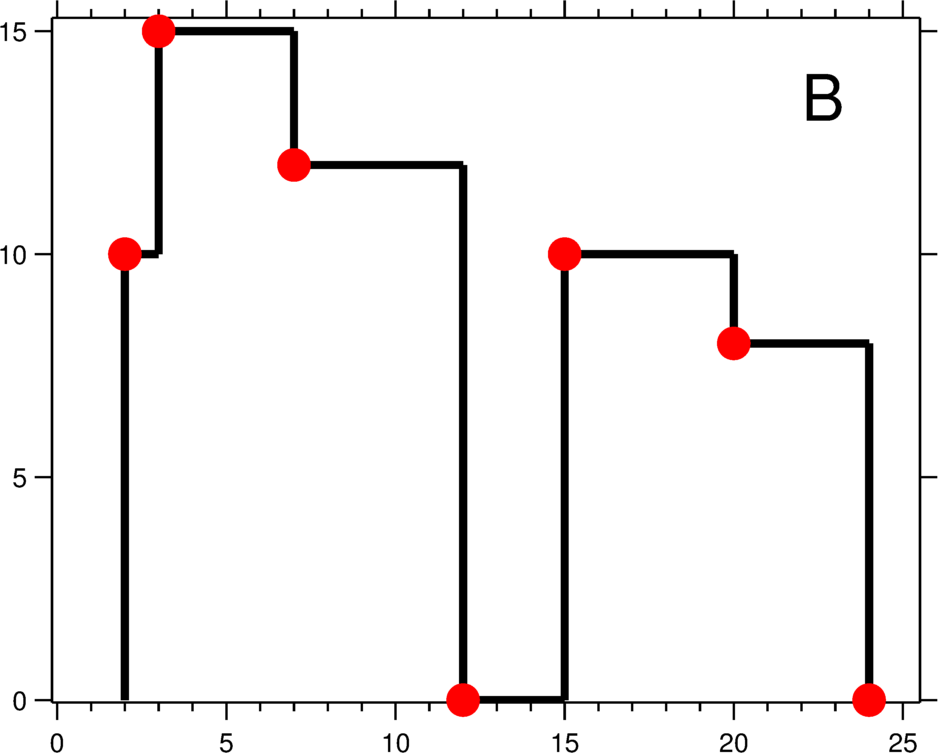1. 如何维护最值。当一个高楼的右边界消失，剩下的各个小楼间还需要选出最大值作为天际线。剩下重重叠叠的小楼很多，树状数组如何维护区间最值是解决此类题的关键。
2. 如何维护天际线的转折点。有些楼与楼并非完全重叠，重叠一半的情况导致天际线出现转折点。如上图中标记的红色转折点。树状数组如何维护这些点呢？

const LEFTSIDE = 1
const RIGHTSIDE = 2

type Point struct {
xAxis int
side  int
index int
}

func getSkyline3(buildings [][]int) [][]int {
res := [][]int{}
if len(buildings) == 0 {
return res
}
allPoints, bit := make([]Point, 0), BinaryIndexedTree{}
// [x-axis (value), [1 (left) | 2 (right)], index (building number)]
for i, b := range buildings {
allPoints = append(allPoints, Point{xAxis: b, side: LEFTSIDE, index: i})
allPoints = append(allPoints, Point{xAxis: b, side: RIGHTSIDE, index: i})
}
sort.Slice(allPoints, func(i, j int) bool {
if allPoints[i].xAxis == allPoints[j].xAxis {
return allPoints[i].side < allPoints[j].side
}
return allPoints[i].xAxis < allPoints[j].xAxis
})
bit.Init(len(allPoints))
kth := make(map[Point]int)
for i := 0; i < len(allPoints); i++ {
kth[allPoints[i]] = i
}
for i := 0; i < len(allPoints); i++ {
pt := allPoints[i]
if pt.side == LEFTSIDE {
bit.Add(kth[Point{xAxis: buildings[pt.index], side: RIGHTSIDE, index: pt.index}], buildings[pt.index])
}
currHeight := bit.Query(kth[pt] + 1)
if len(res) == 0 || res[len(res)-1] != currHeight {
if len(res) > 0 && res[len(res)-1] == pt.xAxis {
res[len(res)-1] = currHeight
} else {
res = append(res, []int{pt.xAxis, currHeight})
}
}
}
return res
}

type BinaryIndexedTree struct {
tree     []int
capacity int
}

// Init define
func (bit *BinaryIndexedTree) Init(capacity int) {
bit.tree, bit.capacity = make([]int, capacity+1), capacity
}

func (bit *BinaryIndexedTree) Add(index int, val int) {
for ; index > 0; index -= index & -index {
bit.tree[index] = max(bit.tree[index], val)
}
}

// Query define
func (bit *BinaryIndexedTree) Query(index int) int {
sum := 0
for ; index <= bit.capacity; index += index & -index {
sum = max(sum, bit.tree[index])
}
return sum
}



## 三. 常见应用 #

### 1. 求逆序对 #

func reversePairs(nums []int) int {
if len(nums) <= 1 {
return 0
}
arr, newPermutation, bit, res := make([]Element, len(nums)), make([]int, len(nums)), template.BinaryIndexedTree{}, 0
for i := 0; i < len(nums); i++ {
arr[i].data = nums[i]
arr[i].pos = i
}
sort.Slice(arr, func(i, j int) bool {
if arr[i].data == arr[j].data {
if arr[i].pos < arr[j].pos {
return true
} else {
return false
}
}
return arr[i].data < arr[j].data
})
id := 1
newPermutation[arr.pos] = 1
for i := 1; i < len(arr); i++ {
if arr[i].data == arr[i-1].data {
newPermutation[arr[i].pos] = id
} else {
id++
newPermutation[arr[i].pos] = id
}
}
bit.Init(id)
for i := 0; i < len(newPermutation); i++ {
res += (i + 1) - bit.Query(newPermutation[i])
}
return res
}


	for i := len(s) - 1; i > 0; i-- {
res += bit.Query(newPermutation[i] - 1)
}


### 2. 求区间逆序对 #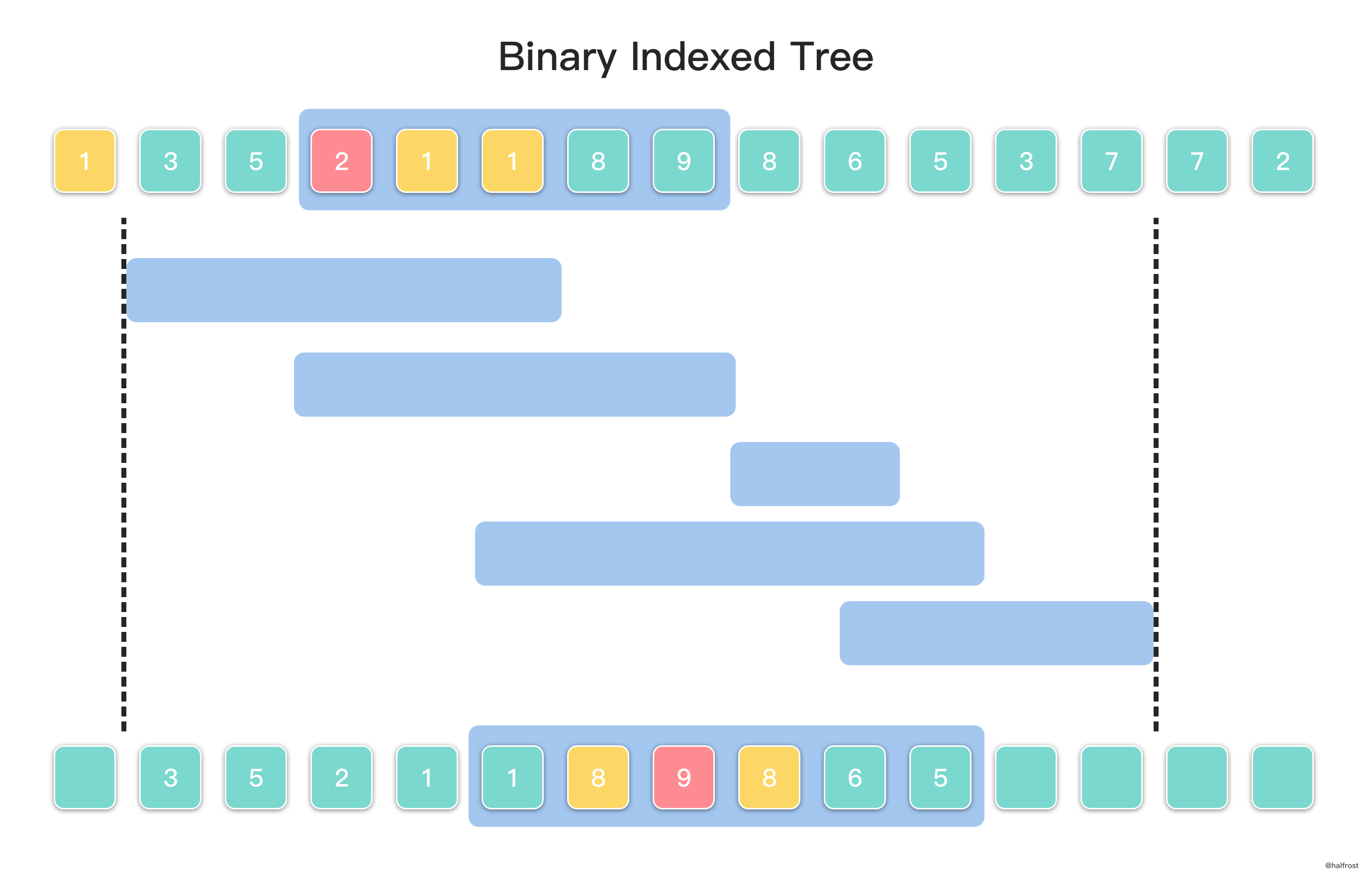\begin{aligned} Query(A[i] - 1) - C[i] &= Query(A - 1) - C \\ &= Query(9 - 1) - C = Query(8) - C\\ &= 9 - 6 = 3\\ \end{aligned}

1. 离散化数组 A[i]
2. 对所有区间按照右端点单调不减排序
3. 按照区间排序后的结果，从左往右依次遍历每个区间。依照从左往右的区间覆盖元素范围，从左往右将 A[i] 插入至树状数组中，每个元素插入之前计算辅助数组 C[i]。
4. 依次遍历每个区间内的所有元素，对每个元素计算 Query(A[i] - 1) - C[i]，累加逆序对的结果即是这个区间所有逆序对的总数。

## 四. 二维树状数组 #

// BinaryIndexedTree2D define
type BinaryIndexedTree2D struct {
tree [][]int
row  int
col  int
}

func (bit2 *BinaryIndexedTree2D) Add(i, j int, val int) {
for i <= bit2.row {
k := j
for k <= bit2.col {
bit2.tree[i][k] += val
k += lowbit(k)
}
i += lowbit(i)
}
}

// Query define
func (bit2 *BinaryIndexedTree2D) Query(i, j int) int {
sum := 0
for i >= 1 {
k := j
for k >= 1 {
sum += bit2.tree[i][k]
k -= lowbit(k)
}
i -= lowbit(i)
}
return sum
}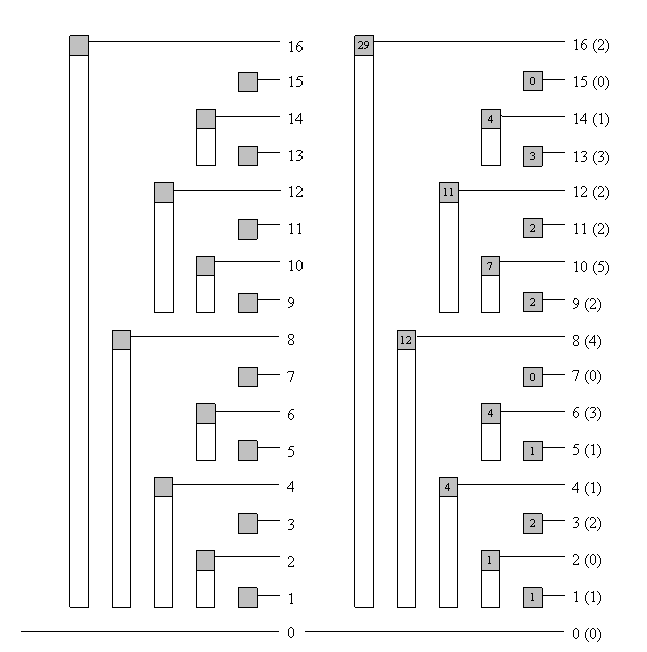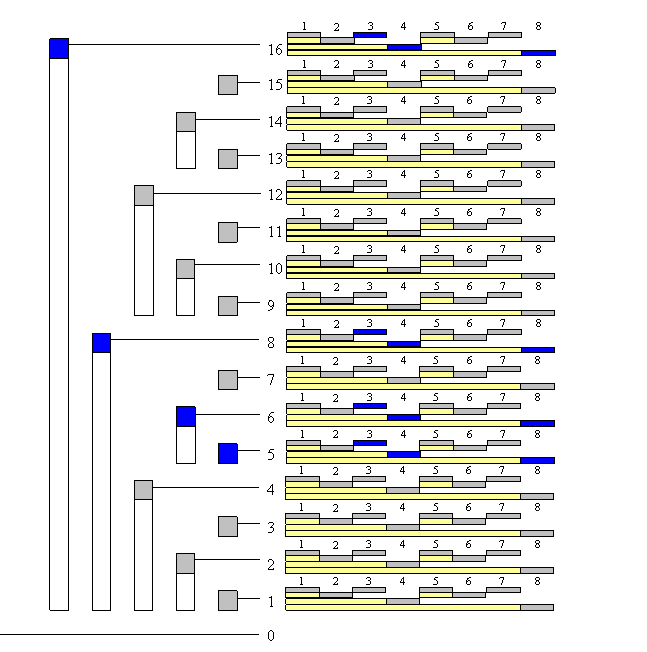Nov 26, 2021Edit this page